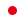﻿中国排球协会 >  机构介绍

一、训练科研委员会

主任委员：李全强

副主任委员：于光岩(中心)、胡进(中心)、陈忠和(福建)、沈富麟(上海)、周建安(四川)、李宗浩(天津体院)、钟秉枢(北体大)

委员：岳伟(辽宁)、李援非(辽宁)、赵勇(辽宁)、李牧(北京)、王宝泉(天津)、唐 凌(河北)、鞠根寅(上海)、张立明(上海)、刘 斌(江苏)、邸安和(江苏)、卢卫中(江苏)、薛永业(江苏)、王志刚(浙江)、王贺兵(浙江)、徐建德(浙江)、肖青松(福建)、韩晓华(山东)、安家杰(山东)、辛春生(山东)、程慎修(河南)、谢国臣(河南)、张仁江(湖北)、张镇民(广东)、杨立群(广东)、孙 彤(云南)、何琦(云南)、张利明(四川)、葛春林(北体大)、谢敏豪(北体大)、金学斌(西安体院)、朱玉青(解放军)、李海运(解放军)、张迪(解放军)、唐磊军(南部)

秘书：李国东(中心)

二、青少年委员会

主任委员：杨立国(教育部)

副主任委员：于光岩(中心)、王晓毅(教育部)、刘国庆(大排协)、张志杰(天津)

委员：贺明(教育部)、刘树平(中排协)、王安利(北体大)、赵立明(北京)、田 争(天津)、李占府(河北)、鲍海涛(辽宁)、张久成(辽宁)、王志峰(上海)、刘海鹏(上海)、江海林(江苏)、杨欣生(江苏)、高 峰(江苏)、吴 胜(浙江)、陈合成(福建)、刘 新(山东)、王嘉琴(山东)、张虎豹(河南)、牛 江(河南)、孟 琦(湖北)、叶文(四川)、林 楠(解放军)、郝振生(内蒙古)

秘书：李国东(中心)

三、俱乐部委员会

主任委员：李全强(中心)

副主任委员：于光岩(中心)

委员：王卫军(北京)、田建君(天津)、常良才(天津)、李焕平(河北)、陆 宏(辽宁)、吕宁馨(上海)、赵善文(江苏)、俞 波(浙江)、滕穗莲(浙江)、叶珠莲(福建)、刘 伟(山东)、张晓阳(河南)、杨赞波(广东)、陈 林(四川)、张玉美(四川)、杨旭光(云南)、董瑞军(解放军)、崔咏梅(解放军)、刘晓宁(南部)

秘书：李国东(中心)

四、竞赛委员会

主任委员：张蓉芳

副主任委员：蔡 毅

委员：张恩祥(天津)、岳伟(辽宁)、张静(上海)、李一冰(江苏)、沈达军(浙江)、侯玉珠(福建)、程慎修(河南)、陈蓉(四川)

秘书： 魏胜凡(中心)

五、裁判委员会

主任委员：张蓉芳

顾问：曲正中(天津体院)、陈玉鑫(江苏)

副主任委员：王玮(河南)、黄锋(四川)、蔡毅(中心)

委员：孙剑辉(北京)、李琛(上海)、孙国民(江苏)、骆文笙(浙江)、刘江(四川)、高子琪(北体大)

秘书：魏胜凡(中心)

六、沙滩排球委员会

主任委员：刘文斌

副主任委员：王建平(中心)、向前(中心)、刘征(辽宁)、杨培刚(上海)、缪志红(上海)

委员：刘宏(教育部学生体协)、陆卫平(北京)、沈广晋(山西)、刘智浩(山西)、徐林胤(上海)、刘斌(江苏)、张希(江苏)、王志刚(浙江)、王雷钧(宁波)、肖青松(福建)、程兆鹤(福建)、颜建明(福建)、韩晓华(山东)、翟成竹(山东)、刘锋(广东)、谭文华(广州)、陈蓉(四川)、杨毅光(海南)、陈导(海南)、龙建贵(新疆)、许永鹏(总政宣传部文体局)、王红英(上海体院)、李军(成都体院)、林群(漳州基地)、王万钧(海埂基地)、刘晋廷(三亚基地)、戚震(耐克公司)

秘书：崔龙

七、新闻委员会

主任委员：李全强

副主任委员：辛沂(中心)、张兴(中央电视台)、王成(宣传司)、魏胜凡(中心)、杨玛俐

委员：曲北林(新华社)、王镜宇(新华社)、汪大昭(人民日报)、李长云(人民日报)、郭璋(中国电视台体育频道)、崔嘉(中央电视台体育频道)、贾博伟(中央电视台国际频道)、王延辉(中央人民广播电台)、苏畅(中国体育报社)、刘亚茹(华奥星空)、李小伟(中国教育报)、龙渊(新浪网)、李晖(北京青年报)

秘书：应可

八、市场开发推广委员会

主任委员：张蓉芳

副主任委员：杨 斌(CCTV-5)、阮伟(中视体育)、吕雅君、魏胜凡

委员：甘铭琪(阿迪达斯)、林宇(益海嘉里粮油)、章杰艺(香港洁福地板)、徐阳(安踏体育)、刘燕(益海嘉里粮油)、季正德(无锡华健)、王飞虎(中国洁福地板)、 赵逸岩(阿迪达斯)、戚震(耐克)、王宏伟(金龙鱼)

秘书：滕秀明

,